King Fahd University of Petroleum and Minerals

Faculty of Science      Per-Year Math Program

Math 002 - Term 032

Recitation hour (5.1 & 5.2)

Please cover at least four questions

Question1

a)     Convertto radian measure.

b)    Convert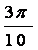radians to degree measure.

Question2

Ifis of the complement of the angleand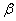is the supplement of the angle, then find the measure of the angle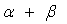.

Question3

a)     Find the smallest positive angle coterminal with the angle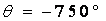.

b)    Find the exact value of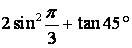Question4

a) Find the length of an arc that subtends a central angle of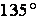in a circle of diameter 40 ft.

b) A wheel is rotating at 200 revolutions per minute .Find the angular speed of the wheel in radian per second.

Question5

Find the height of a building if the angle of elevation to the top of the building changes from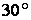to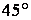as the observer moves a distance of 80 ft toward the building.